# Lines and Angles Class 9

## CBSE Class 9 Maths Lines and Angles Notes:-

Lines and Angles for class 9 notes are provided here. The chapter lines and angles for Class 9 demonstrates the two important shapes in Geometry. Most of these shapes are formed by the combination of lines and angles. A line is a set of points that has length but no width, whereas an angle is formed by joining two rays at a common point called the vertex. There are different types of lines and angles introduced in geometry which we are going to learn here. The notes on lines and angles cover various concepts such as parallel lines, transversal, angles, intersecting lines, interior angles along with the examples. Go through the article to learn about lines and angles.

Also read: Lines and Angles Introduction

## Basic Terms and Definitions on Lines and Angles

Line Segment: A line that has two endpoints is called a line segment.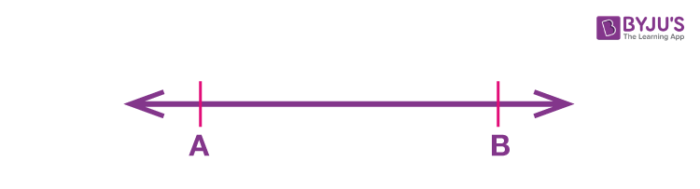Ray: A line with one endpoint and the other end of the line extending up to infinity is called a ray.Collinear points: When three or more points lie on the same line, they are said to be collinear.

Non-collinear points: When three or more points do not lie on the same line, they are non-collinear.

Angle: An angle is formed by two rays meeting at a common point (called a vertex), and the rays forming the angle are called arms of the angle.

Acute Angle: An angle that measures between 0° and 90° is called an acute angle.

Obtuse angle: An angle that measures between 90° and 180° is called an obtuse angle.

Right angle: An angle that is equal to 90° is called a right angle.

Reflex angle: An angle greater than 180° but less than 360° is called a reflex angle.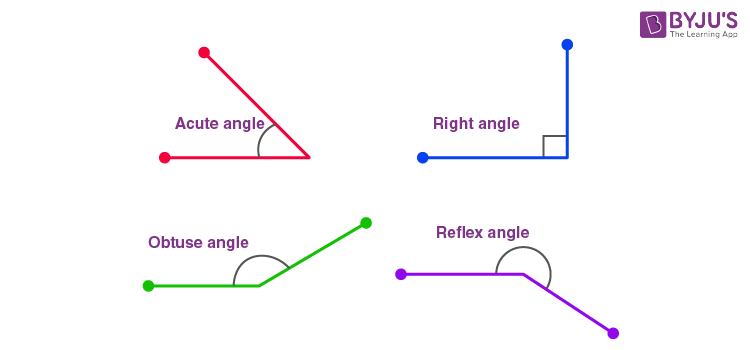Complementary angles: When sum of two angles is equal to 90°.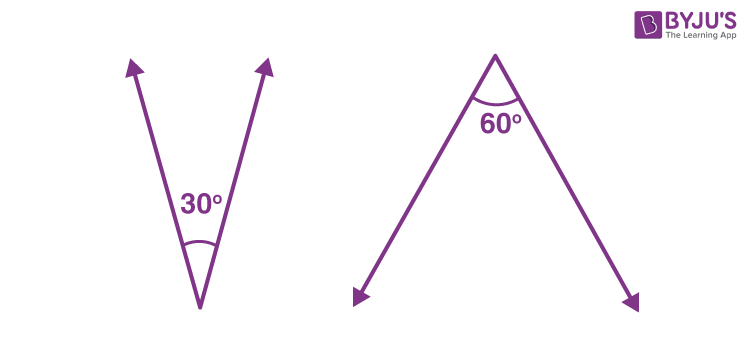Supplementary angles: When sum of two angles is equal to 180°.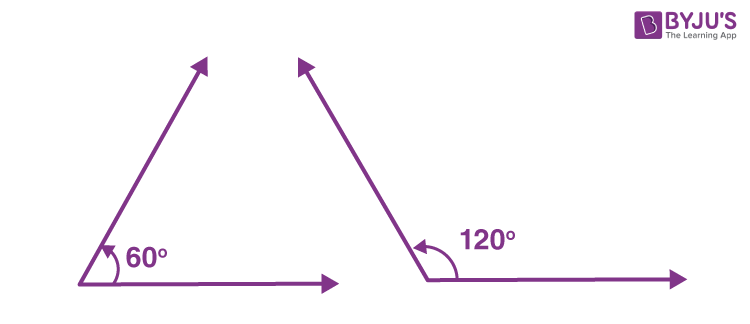Also read: Supplementary And Complementary Angles

Adjacent angles: Two angles with a common vertex, a common arm and their non-common arms on different sides of the common arm.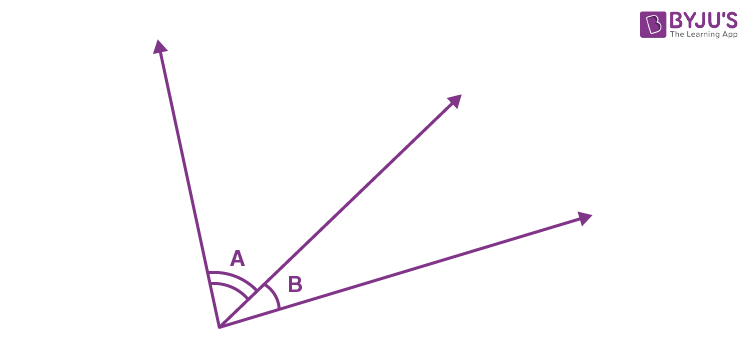Linear pairs of angles: When 2 adjacent angles are supplementary, i.e. they form a straight line (add up to 180∘), they are called a linear pair.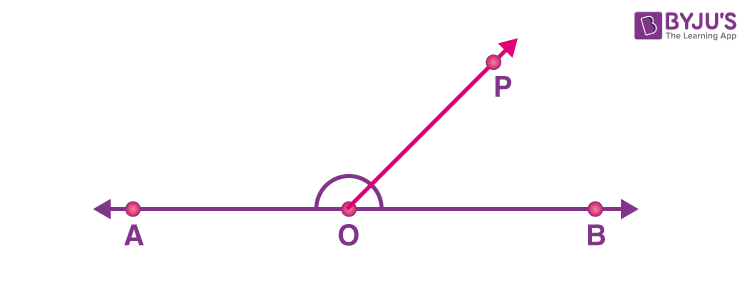Vertically opposite angles: When two lines intersect at a point, they form equal angles that are vertically opposite to each other.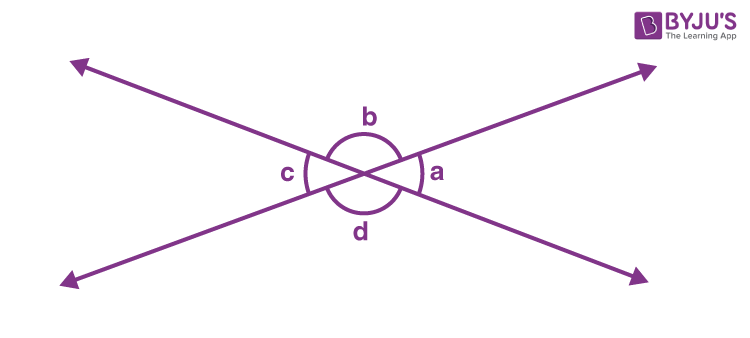Also read: Lines And Angles – Basic Terms

## Intersecting and Non-Intersecting Lines

• When two lines intersect each other at a common point, they are said to be intersecting lines.
•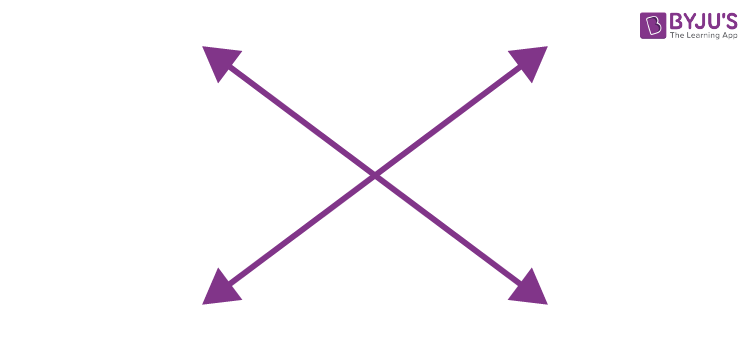• Non-intersecting lines are parallel lines that do not intersect each other at a common point.
•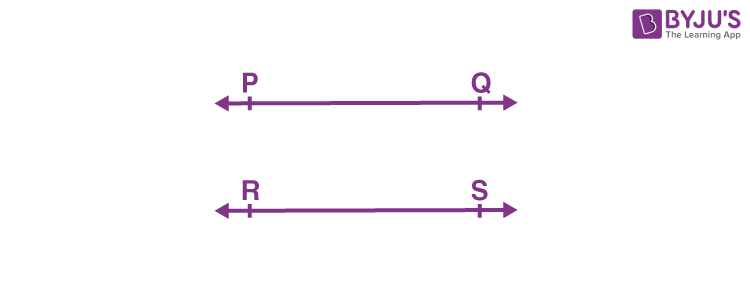#### For more information on Parallel Lines and Intersecting Lines, watch the below video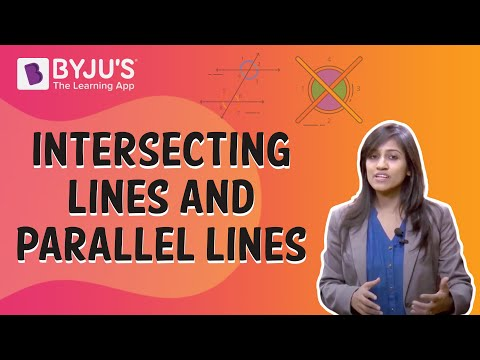## Pairs of Angles

Above, we have learned the different types of pairs of angles, such as:

• Complementary angles
• Supplementary angles
• Linear pair of angles

Let us discuss the axioms related to all these pairs of angles.

### Axiom – Linear Pair of Angles

If a ray stands on a line, the sum of two adjacent angles so formed is 180°.

### Axiom – Converse of Linear Pair of Angles

If the sum of two adjacent angles is 180°, the non-common arms of the angles form a line.

### Theorem – Vertically Opposite Angles

If two lines intersect each other, the vertically opposite angles are equal.

### Parallel lines with a transversal

A line that intersects two or more lines is called a transversal.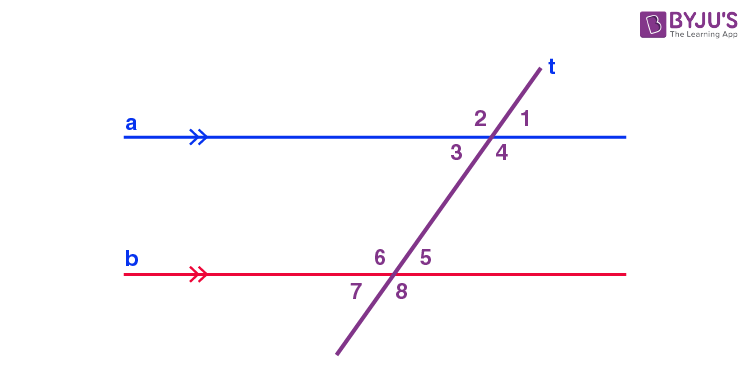• ∠1=∠5,∠2=∠6,∠4=∠8 and ∠3=∠7(Corresponding angles)
• ∠3=∠5,∠4=∠6 (Alternate interior angles)
• ∠1=∠7,∠2=∠8 (Alternate exterior angles)

Interior angles on the same side of the transversal are referred to as consecutive interior angles, allied angles, or co-interior angles.

### Corresponding angles axiom

If a transversal intersects two parallel lines, then each pair of corresponding angles are equal.

### Converse of corresponding angles axiom

If a transversal intersects two lines such that a pair of corresponding angles are equal, then the two lines are parallel to each other.

### Theorem – Alternate interior angles

If a transversal intersects two parallel lines, then each pair of alternate interior angles are equal.

### Theorem – Converse of alternate interior angles

If a transversal intersects two lines such that a pair of alternate interior angles are equal, then the two lines are parallel.

### Theorem – Sum of co-interior angles is supplementary

If a transversal intersects two parallel lines, then each pair of interior angles on the same side of the transversal is supplementary.

### Theorem – Converse of the sum of co-interior angles is supplementary

If a transversal intersects two lines such that a pair of interior angles on the same side of the transversal are supplementary, then the two lines are parallel.

## Lines parallel to the same line

Lines that are parallel to the same line are also parallel to each other.

### Theorem – Lines Parallel to the Same Line

Lines which are parallel to the same line are parallel to each other.

## Angle Sum Property of a Triangle

Theorem 1: The sum of the angles of a triangle is 180º.
Theorem 2: If a side of a triangle is produced, then the exterior angle so formed is equal to the sum of the two interior opposite angles.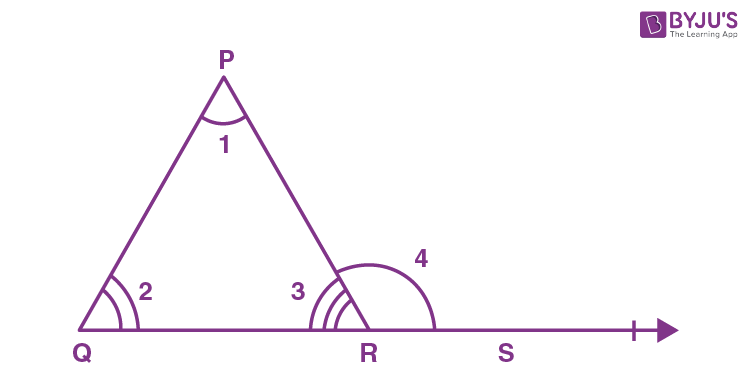∠4=∠1+∠2

#### For more information on Properties Of Triangle, watch the below videoTo know more about Properties of a Triangle, visit here.